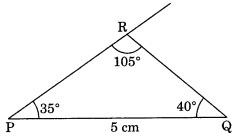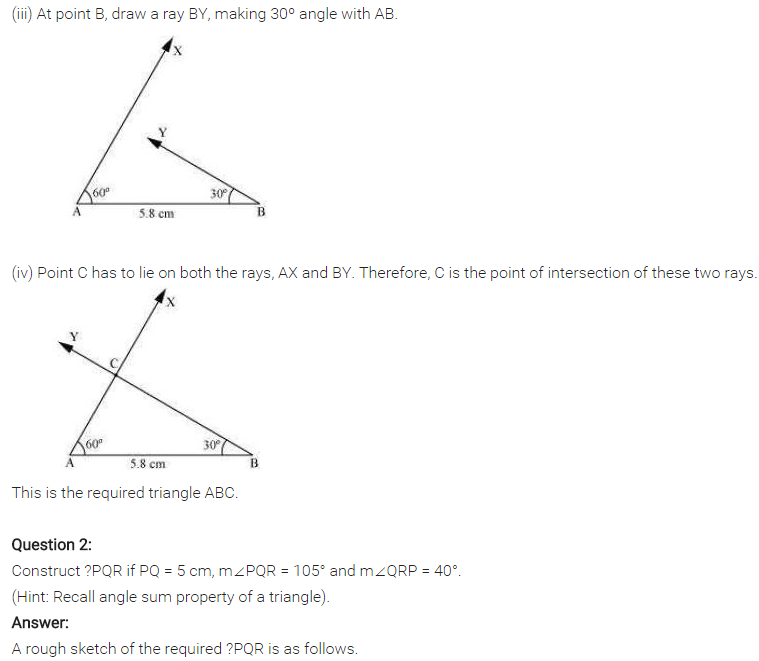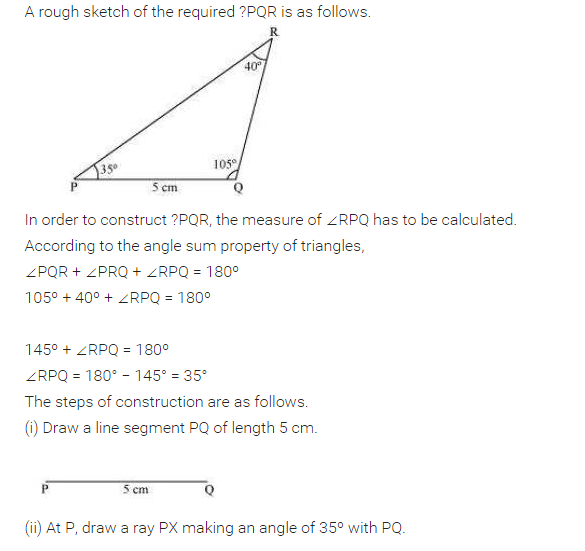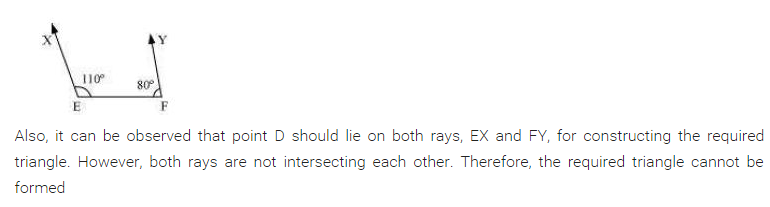# NCERT Solutions for Class 7 Maths Chapter 10 Practical Geometry Ex 10.4

NCERT Solutions for Class 7 Maths Chapter 10 Practical Geometry Ex 10.4

### NCERT Solutions for Class 7 Maths Chapter 10 Practical Geometry Ex 10.4

NCERT Solutions for Class 7 Maths Chapter 10 Practical Geometry Exercise 10.4
Ex 10.4 Class 7 Maths Question 1.
Construct ∆ABC, given m ∠A = 60°, m ∠B = 30° and AB = 5.8 cm.Solution:
Steps of construction:
(i) Draw AB = 5.8 cm.
(ii) Draw ∠A = 60° and ∠B = 30° to meet each other at C.
(iii) ABC is the required triangle.
Using ASA criterion

Ex 10.4 Class 7 Maths Question 2.
Construct ∆PQR if PQ = 5 cm, m ∠PQR = 105° and m∠QRP = 40°.
(Hint: Recall angle-sum property of a triangle)
Solution:
Given: m∠PQR = 40°, m∠QRP = 105°
∵ m∠PQR + m∠QRP + ∠QPR = 180° (Angle sum property of a triangle)
105° + 40° + ∠QPR = 180°
145° + ∠QPR = 180°
∴ ∠QPR = 180° – 145° = 35°Steps of construction:
(i) Draw PQ = 5 cm.
(ii) Draw ∠QPR = 35° and ∠PQR = 40° to meet each other at R.
(iii) ∆PQR is the required triangle.
[Using AAS or ASA criterion]

Ex 10.4 Class 7 Maths Question 3.
Examine whether you can construct ∆DEF such that EF = 7.2 cm, m∠E = 110° and m∠F = 80°. Justify your answer.
Solution:
To construct ∆DEF with the given measurement, is not possible.
∵ m∠E + m∠F = 110° + 80°
= 190° > 180° [Sum of the angles of a triangle = 180°]
∴ ADEF is not possible to construct.## SabDekho

The Complete Educational Website